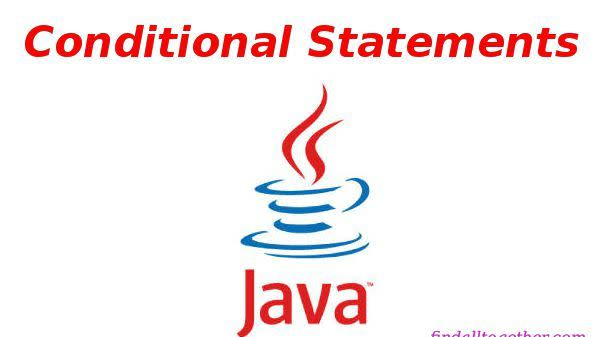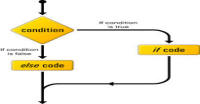# Conditional statements in Java

Conditional statements check conditions and take decision what to to.

### If Statements:

If we want that a code execute if a condition satisfy otherwise not than we use if statements. For examples, we are taking an integer from console and want to print 'number is between 0 and 100' if number is between 0 and 100.

```package flowkl;

import java.util.Scanner;
public class Tutorials
{
public static void main(String args[])
{
Scanner input = new Scanner(System.in);
System.out.print("type an integer value: ");
int a = input.nextInt();
// check for condition
// condition returns true or false
if (a > 0 && a <= 100)
{
System.out.print("a is between 0 and 100 (inclusive)");
}
}
}
```

Run this code and enter different values.

### If else statements:

If else statement provides an alternative. If condition fails then alternative code will run. In above example if we want to print 'number is outside the range' for numbers which are not between 0 and 100 then we will use else statement.

```package flowkl;

import java.util.Scanner;
public class Tutorials
{
public static void main(String args[])
{
Scanner input = new Scanner(System.in);
System.out.print("type an integer value: ");
int a = input.nextInt();
// check for condition
// condition returns true or false
if (a > 0 && a <= 100)
{
System.out.print("a is between 0 and 100 (inclusive)");
}
else
{
System.out.print("number is outside the range");
}
}
}
```

### else if Statements:

It provides more alternatives. For example, in above example if we want to print for multiple ranges then code will be:

```package flowkl;

import java.util.Scanner;
public class Tutorials
{
public static void main(String args[])
{
Scanner input = new Scanner(System.in);
System.out.print("type an integer value: ");
int a = input.nextInt();
// check for condition
// condition returns true or false
if (a > 0 && a <= 100)
{
System.out.print("a is between 0 and 100 (inclusive)");
}
else if (a > 100 && a <= 200)
{
System.out.print("a is between 100 and 200 (inclusive)");
}
else if (a > 200 && a <= 300)
{
System.out.print("a is between 200 and 300 (inclusive)");
}
else if (a > 300 && a <= 400)
{
System.out.print("a is between 300 and 400 (inclusive)");
}
else
{
System.out.print("number is outside the range");
}
}
}
```

### Nested statements:

We can write if or if else or else if statements inside if or else or else if statements.

```package flowkl;

import java.util.Scanner;
public class Tutorials
{
public static void main(String args[])
{
Scanner input = new Scanner(System.in);
System.out.print("type an integer value: ");
int a = input.nextInt();
// check for condition
// condition returns true or false
if (a > 0 && a <= 100)
{
if (a > 0 && a <= 50)
{
System.out.print("a is between 0 and 50 (inclusive)");
}
else
{
System.out.print("a is between 50 and 100 (inclusive)");
}
}
else if (a > 100 && a <= 200)
{
if (a > 100 && a <= 150)
{
System.out.print("a is between 100 and 150 (inclusive)");
}
else if (a > 150 && a <= 200)
{
System.out.print("a is between 150 and 200 (inclusive)");
}
}
else if (a > 200 && a <= 300)
{
System.out.print("a is between 200 and 300 (inclusive)");
}
else if (a > 300 && a <= 400)
{
System.out.print("a is between 300 and 400 (inclusive)");
}
else
{
System.out.print("number is outside the range");
}
}
}
```

### Switch Statements:

Switch statements are useful when we have to compare a single variable with a list of variables and decide which code has to be execute.

Syntax of switch statements is:

```switch(variable)
{
case 1:
//code
break;
case 2:
//code
break;
...
...
...
case n:
//code
break;
default:
break;
}
```
• Every case is compared with variable. If any case matches with variable then code inside that case (until break statement) will run.
• Once break statement is executed, switch will stop executing code and program will come out from the switch statement.
• Cases must be of same data types that of variable like int.
• Variable can only be integer, short, byte or char.
• Every switch statement must has a default case. Break statement after default may be neglected because there is no case after that case.
• NOTE: if you forget break statement then code of next case will also be executed.

Lets take a very simple example: we have to input an integer number and program will print if number is between 1-3 or between 4-7 or 8 or 9 or 10 or outside the range.

```package flowkl;

import java.util.Scanner;
public class Tutorials
{
public static void main(String args[])
{
Scanner input = new Scanner(System.in);
System.out.print("type an integer value: ");
int number = input.nextInt();

// write a switch statement
switch(number)
{
// check for 1 to 3
// no break statement require because we want to execute same code
case 1:
case 2:
case 3:
System.out.println("number is between 1 to 3");
// break switch statement
break;
// check for 4 to 7
case 4:
case 5:
case 6:
case 7:
System.out.println("number is between 4 to 7");
// break switch statement
break;
// check for 8
case 8:
System.out.println("number is 8");
// break switch statement
break;
case 9:
System.out.println("number is 9");
// break switch statement
break;
case 10:
System.out.println("number is 10");
// break switch statement
break;
default:
System.out.println("number is outside the range");
}

}
}
```

It is your exercise to implement the example used in nested statement using switch statement after reading this section.

HINT: Divide number by 50.

### Exercise:

Take a variable as mark and print the grade according to this table using both if else and switch statements.

 Min marks Max marks Grade 0 30 F 31 40 E 41 50 D 51 60 C 61 70 C+ 71 80 B+ 81 90 A 91 100 A+

#### Click on banner below to Learn: PHP with Laravel for beginners - Become a Master in LaravelHarish, a technical core team member at www.lyflink.com with five year experience in full stack web and mobile development, spends most of his time on coding, reading, analysing and curiously following businesses environments. He is a non-graduate alumni from IIT Roorkee, Computer Science and frequently writes on both technical and business topics.

### Related ArticlesPHP stands for PHP: Hypertext Preprocessor. PHP is known as a server side scripting and interpreter language for web ...
##### Introduction to PHPIn this tutorial, we will study about conditional statements (if else statements and switch statements). These statem...
##### Conditional statements in phpIn this tutorial, we will study about strings and operations upon strings. Due to long length of this section, I have...

### The Complete Web Developer Course - Build 25 Websites